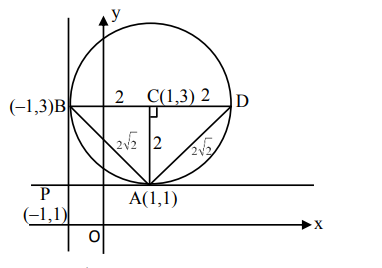# Two tangents are drawn from the point`
Question:

Two tangents are drawn from the point $\mathrm{P}(-1,1)$ to the circle $x^{2}+y^{2}-2 x-6 y+6=0$. If these tangents touch the circle at points $A$ and $B$, and if $D$ is a point on the circle such that length of the segments $\mathrm{AB}$ and $\mathrm{AD}$ are equal, then the area of the triangle ABD is equal to:

1. 2

2. $(3 \sqrt{2}+2)$

3. 4

4. $3(\sqrt{2}-1)$

Correct Option: , 3

Solution:$\triangle \mathrm{ABD}=\frac{1}{2} \times 2 \times 4$

$=4$